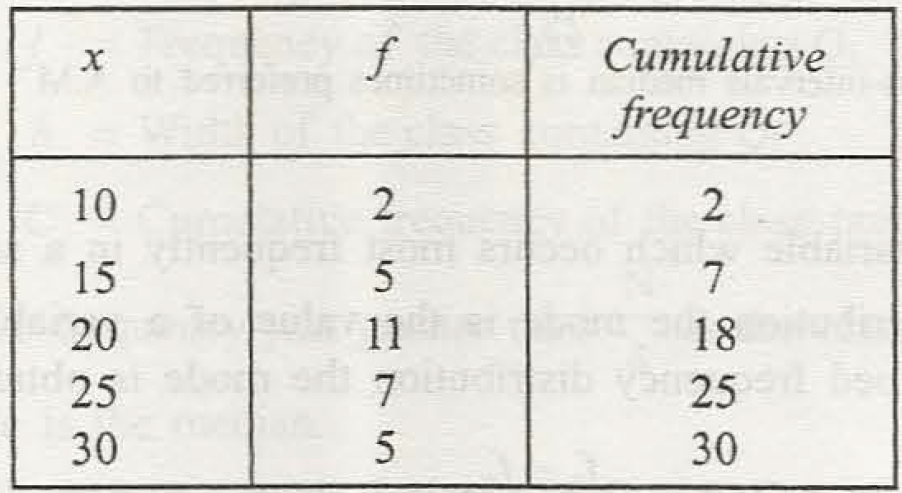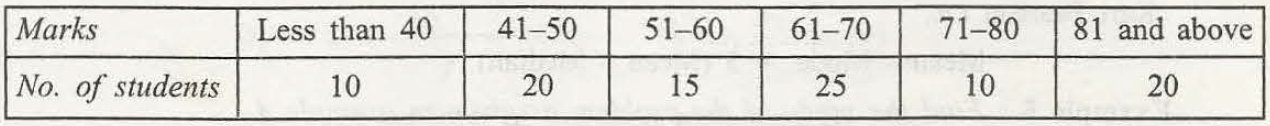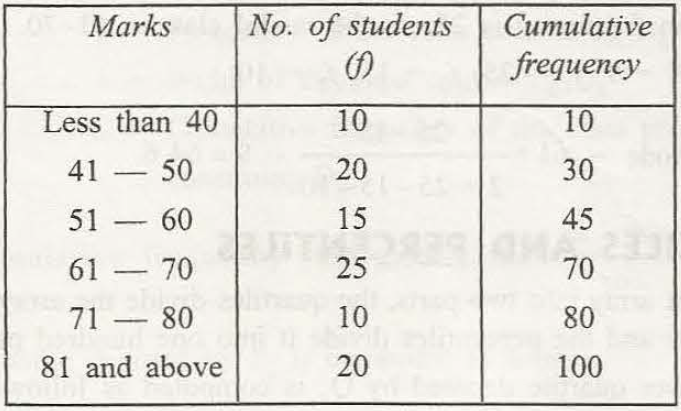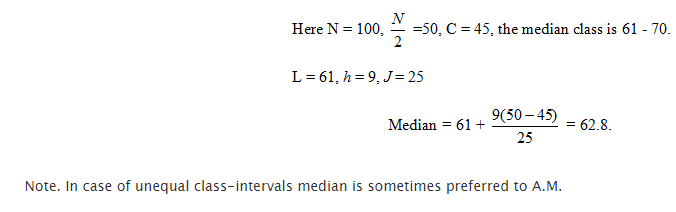Median is an important term in Statistics. Any student can easily understand this term if he considers it as the middle value. However, there are three different ways to find out median. These are as follows –

1. The perfect term median means the middle term. However, the numbers provided must be arranged either in ascending order or in descending order. Now, if there are some numbers as 6, 9, 12, 13, 11, 10 and 15, then the first step of getting the exact median is to arrange the numbers in ascending or descending manner.

Let us arrange in ascending order. Now, it becomes 6, 9, 10, 11, 12, 13 and 15. The middle number is 11 and this is known as the median of this set of numbers. A student can easily find out the middle number as median, because it is a set of odd numbers.

Sometimes the numbers are the set of even numbers as 12, 16, 14, 18, 11 and 15. At this time, 11, 12, 14, 15, 16 and 18 is the exact arrangement in the ascending manner. So, in this arranged way the median can easily be found by adding 2 numbers in the middle as (14 + 15)/2 = 14.5.1. Simple frequency distribution needs proper median and for that the exact value is the next number larger than the cumulative frequency distribution. If the total number N is 40, then cumulative frequency = 40/2 = 20.

Now, it is important to point out the value just greater than 20. So, it may be 21 or 22 or any particular number greater than 20 in the frequency list. Now, what is the relative variable value. That exact variable is the median.

1. Distribution for the grouped frequency can easily be determined  by an exact formula as Median = L + h (N/2  – C)/f

L = Lower limit or the boundary

h= class interval width

f = median class frequency

N= total frequency

C = Cumulative frequency

Example 4. Find the median of the following data:Solution.Now, it is clear that how to find out the median in various ways.### Customer Reviews

My Homework Help
Rated 5.0 out of 5 based on 510 customer reviews at